Courses

# Lexical Analysis ( Advance Level) - 1

## 15 Questions MCQ Test Question Bank for GATE Computer Science Engineering | Lexical Analysis ( Advance Level) - 1

Description
This mock test of Lexical Analysis ( Advance Level) - 1 for Computer Science Engineering (CSE) helps you for every Computer Science Engineering (CSE) entrance exam. This contains 15 Multiple Choice Questions for Computer Science Engineering (CSE) Lexical Analysis ( Advance Level) - 1 (mcq) to study with solutions a complete question bank. The solved questions answers in this Lexical Analysis ( Advance Level) - 1 quiz give you a good mix of easy questions and tough questions. Computer Science Engineering (CSE) students definitely take this Lexical Analysis ( Advance Level) - 1 exercise for a better result in the exam. You can find other Lexical Analysis ( Advance Level) - 1 extra questions, long questions & short questions for Computer Science Engineering (CSE) on EduRev as well by searching above.
QUESTION: 1

### In a two pass assembler pseudo code EQU is to be evaluated during

Solution:

In 2-pass assembler during pass-1 pseudo code EQU is to be evaluated.

QUESTION: 2

### Pick the functions which are completely performed in pass 1

Solution:

Operation performed during pass-1 in 2-pass compiler:
1. Processing the DB pseudo-op.
2. Updating the location counter.
3. Processing of EQU pseudo-op.
4. Processing of DS pseudo-op.

QUESTION: 3

### in a context-free grammar

Solution:

The context free grammar is represented as
S → X

where S∈ V(Single symbol non-terminal)

D → (V ∪ T ) * (Any number of terminal and nonterminals)

QUESTION: 4

If two finite state machines M and N are isomorphic, then

Solution:
QUESTION: 5

In an incompletely specified automata

Solution:

In incomplete automata some states have no transition on some tokens.

QUESTION: 6

Consider the following issues:
1. Simplify the phases.
2. Compiler efficiency is improved.
3. Compiler works faster.
4. Compiler portability is enhanced.

Which is/are true in context of lexical analysis?

Solution:

Lexical analysis consists of the phases that
(i) Simplify the subsequent phases by the help of tokens instead of arbitrary elements.
(ii) Since interrelated elements are converted to stream of tokens hence compiler efficiency is improved as no arbitrary storage or value is to be checked independently.
(iii) Computer portability is enhanced.

QUESTION: 7

Which of the following is not generated by the grammar G?

Solution: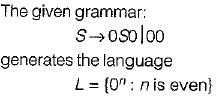QUESTION: 8

Given two DFA’s M1, and M2. They are equivalent if

Solution:

Given two DFA’s M1 and M2. They are equivalent if the language generated by them is same i,e, L(M1) = L(M2)

QUESTION: 9

For the string aabbaa give the derivation tree using grammar:

G = {{S, A}, {a, b}, {S → aAS, S→ a, A → SbA, A→  SS, A → ba}, S}

Solution:

S → aAS, S→ a, A → SbA, A→  SS, A → ba}, S

Input: aabbaa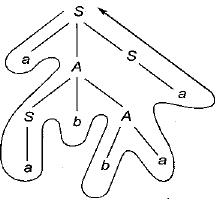QUESTION: 10

Consider a program P that consists of two source modules M1 and M2 contained in two different files. If M1 contains a reference to a function defined in Mthe reference will be resolved at

Solution:

QUESTION: 11

Consider line number 3 of the following C-program.
int main ()  { /* Line 1 */
int i, n; /* Line 2 */
fro (i = 0, i < n, /+ + ); /* Line 3 */
}

Solution:

Error is underlined function fro()
Which is a syntactical error rather than lexical.

QUESTION: 12

Consider the following grammars: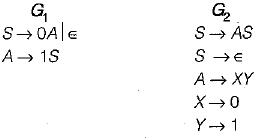Which of the following statements is not true?

Solution:

Here grammar, G2 is not ambiguous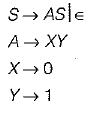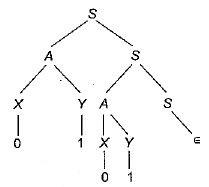Because there exist only one parse tree.

QUESTION: 13

Hash tables can contribute to an efficient average case solution for all of the following problems described below except

Solution:

Hash table can contribute to an efficient average case solution for symbol table look up problem

QUESTION: 14

Consider the grammar: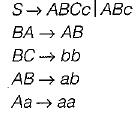Which of the following sentence can be derived by this grammar?

Solution:

S — ABc — abc

QUESTION: 15

Which of the following is not a regular grammars?

Solution:

Mixup notation of left linear and right linear grammar is not allowed in regular grammar.